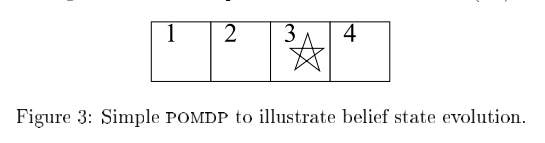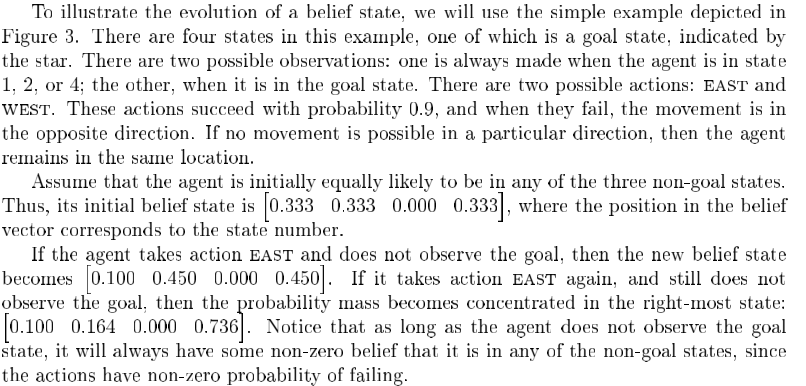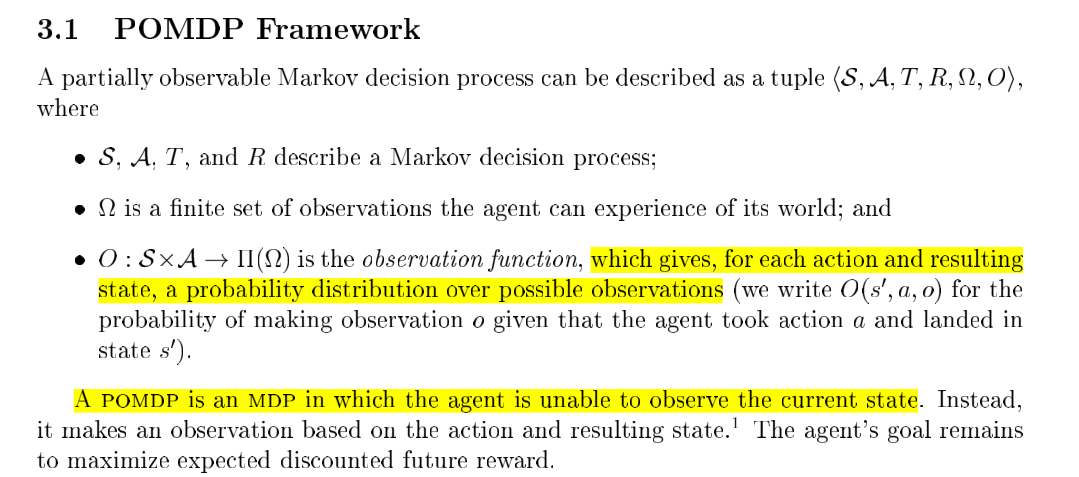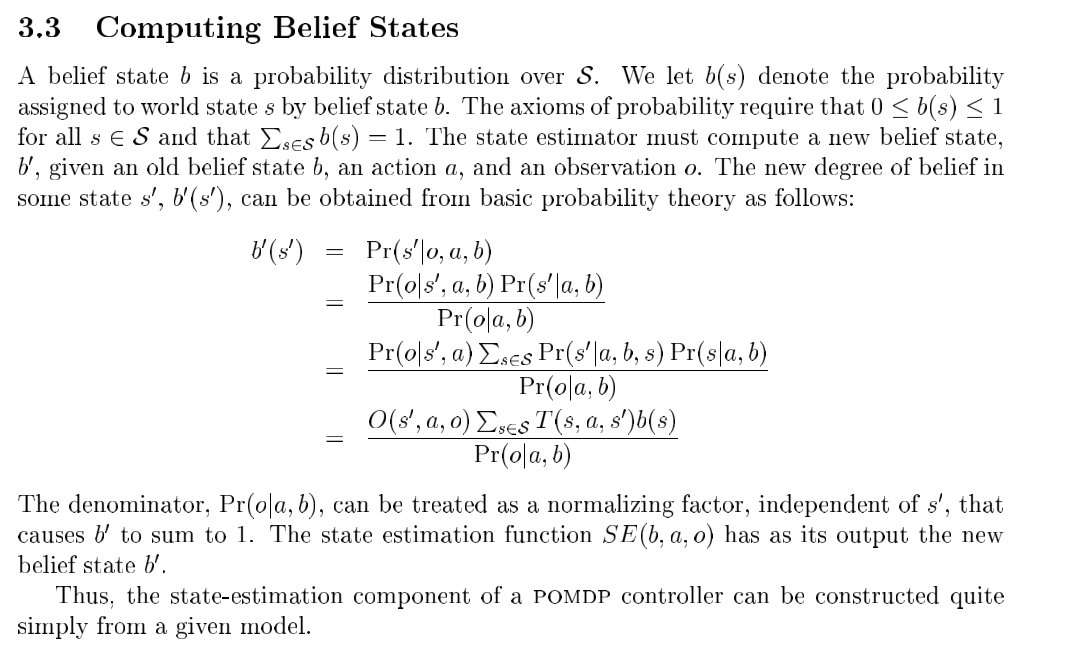WHCSRL 技术网

# POMDP中的观测量(O)和状态量(S)1. 处于状态1的概率：agent 在 t = 0 t=0 时刻处于状态1且采取action失败，或者agent在 t = 0 t=0 时刻处于状态2，且采取action失败： p 1 ( 1 ) = p 0 ( 1 ) ⋅ p A ( 0 ) + p 0 ( 2 ) ⋅ p A ( 0 ) = 1 3 ⋅ 0.1 + 1 3 ⋅ 0.1 = 0.2 3 p_1(1)=p_0(1)\cdot p_A(0)+p_0(2)\cdot p_A(0)=\dfrac13\cdot 0.1+\dfrac13\cdot0.1=\dfrac{0.2}{3}
2. 同理，处于状态2的概率： p 1 ( 2 ) = p 0 ( 1 ) ⋅ p A ( 1 ) + p 0 ( 3 ) ⋅ p A ( 0 ) = 1 3 ⋅ 0.9 + 0 ⋅ 0.1 = 0.9 3 p_1(2)=p_0(1)\cdot p_A(1)+p_0(3)\cdot p_A(0)=\dfrac13\cdot 0.9+0\cdot 0.1=\dfrac{0.9}{3}
3. 处于状态3的概率： p 1 ( 3 ) = p 0 ( 2 ) ⋅ p A ( 1 ) + p 0 ( 4 ) ⋅ p A ( 0 ) = 1 3 ⋅ 0.1 + 1 3 ⋅ 0.1 = 1 3 p_1(3)=p_0(2)\cdot p_A(1)+p_0(4)\cdot p_A(0)=\dfrac{1}{3}\cdot 0.1+\dfrac{1}{3}\cdot 0.1=\dfrac{1}{3}
4. 处于状态4的概率： p 1 ( 4 ) = p 0 ( 3 ) ⋅ p A ( 1 ) + p 0 ( 4 ) ⋅ p A ( 0 ) = 0.2 3 p_1(4)=p_0(3)\cdot p_A(1)+p_0(4)\cdot p_A(0)=\dfrac{0.2}{3}
5. 由于观测到 p 1 ( 3 ) = 0 p_1(3)=0 ，因此状态比值为 2 : 9 : 0 : 9 2:9:0:9 ，即belief state为 [ 0.1 , 0.45 , 0 , 0.45 ] [0.1,0.45,0,0.45]O ( s ′ , a , o ) O(s',a,o) 表示已知agent t + 1 t+1 时刻处于状态 s ′ s' t t 时刻采取动作 a a t + 1 t+1 时刻观察量为 o o 的概率。在例子中一个状态唯一确定一个观察值。例如，当agent处于状态 1 , 2 , 4 1,2,4 时，观察到的一定时未处于目标状态，相反，当agent处于状态 3 3 时，观察到的就一定属于目标状态。